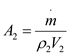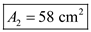# Air enters a nozzle steadily at 2.21 kg/m3 and 40 m/s and leaves at 0.762 kg/m3 and 180 m/s. If the inlet area of the nozzle is 90 cm2, determine (a) the mass flow rate through the nozzle, and (b) the exit area of the nozzle.

Question-AnswerCategory: Fluid MechanicsAir enters a nozzle steadily at 2.21 kg/m3 and 40 m/s and leaves at 0.762 kg/m3 and 180 m/s. If the inlet area of the nozzle is 90 cm2, determine (a) the mass flow rate through the nozzle, and (b) the exit area of the nozzle.

Air enters a nozzle steadily at 2.21 kg/m3 and 40 m/s and leaves at 0.762 kg/m3 and 180 m/s. If the inlet area of the nozzle is 90 cm2, determine (a) the mass flow rate through the nozzle, and (b) the exit area of the nozzle.

Step: 1

Given data
The density at the inlet of the nozzle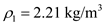The velocity at the inlet is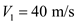The inlet areaThe density at the outlet of the nozzle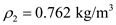The velocity at the outlet is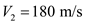Step: 2

(a)
The mass flow rate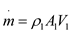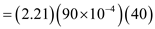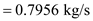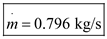Step: 3

(b) The exit area of the nozzle
Since the process is steady mass flow rate remains constant in the nozzle, the exit area can be determined as